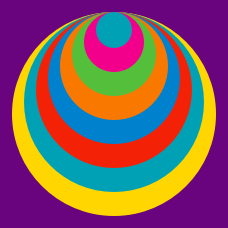Geometry

# Composite Figures - Problem Solving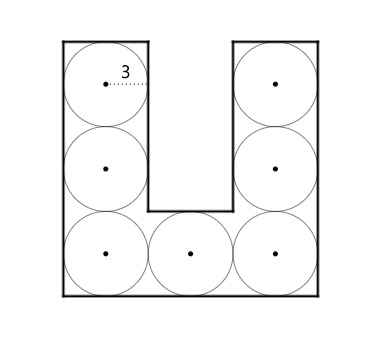The shape above is filled with 7 identical circles with radius 3. What is the perimeter of the shape?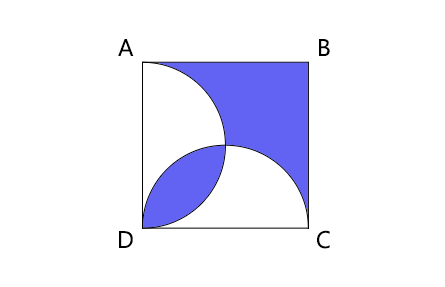$\square{ABCD}$ is a square in which 2 semicircles are overlapping. If $\overline{DC}=2$, what is the perimeter of the colored region?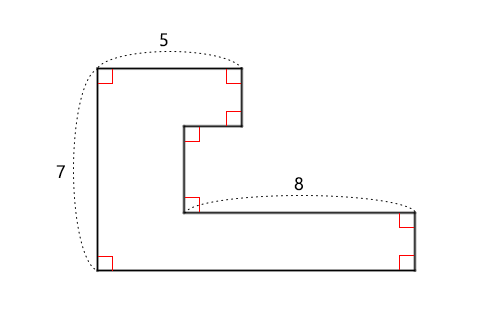What is the perimeter of the shape above?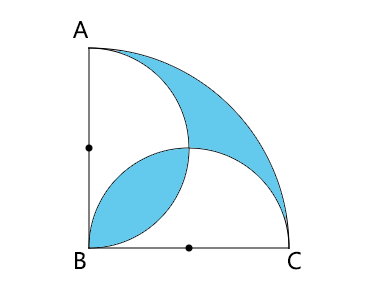In the above diagram, 2 semicircles are overlapping in a quarter circle with radius $\overline{BC}=4$. What is the area of the colored region?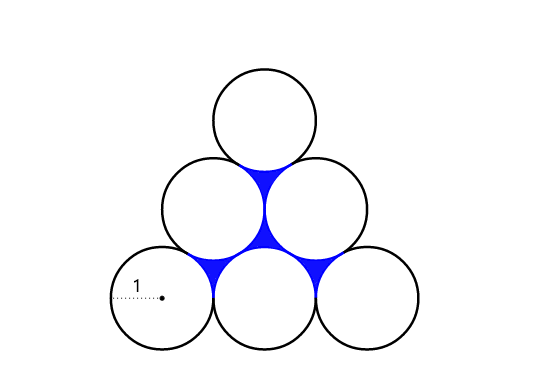6 identical circles with radius 1 are piled as above. What is the perimeter of the colored region?

×